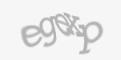0
0
0
s2sdefault

For some, expecially those "in-the-know", calculating a sine wave is a piece of cake. I sure can remember much of math class way back in high school, and remember actually calculating and graphing out some sine and cosine waves. Problem was, that was forever ago, and my Google-Fu only resulted in sine wave calculations at a level FAR beyond my limited understanding of math. Eventually I was able to figure it out, and wish to share my findings with you all.

As part of another little project, I want to "pulse" an LEDin a wave-type up-down pattern, rather than a linear +/- 1 up-down pattern. After weeks of seraching the interwebs for some kind of information I could understand, I eventually came up with the following bit of code, pieced together from a few different sources:

`#!/usr/bin/python`
`from math import *`

`Fs=800`
`f=10`
`i=0`

`while True:`
`    print sin(2*pi*f*i/Fs)`
`    i+=1`

While the results printed out were mostly all good, I would get some very odd results thrown in to the mix similar to the following:

`4.49154267416e-131.70011504358e-123.42657325961e-12-1.27730394862e-126.4039922518e-12-4.25472294081e-122.10545362982e-12-7.23214193301e-125.08287262201e-12-2.93360331102e-128.06029161421e-12-5.91102230321e-12-3.51420462197e-12-1.61248368122e-12-5.36785629772e-13`

And so I changed up the script, and changed the calculation, and changed the way the results were printed to the console, all to no avail... No matter what I did, I either broke the sine wave calculating altogether, or got similar type results. After weeks of poking around with that bit of script, I finally start asking some of my coworkers, "Who has any skills/knowledge with math?" As it turns out, I simply just did not identify/recognize that these numbers were in a scientific notation, meaning that 5.08287262201e-12 is actually equal to 0.00000000000508287262201 (very VERY close to zero); so if we simply round those numbers off, we should get '0'.

`print round( sin(2*pi*f*i/Fs), 0 )`

Indeed, this addition produces the expected results, and the scientific notation is replaced by '0'.

EXCELLENT!

Now, there are some details about the wave and data points that I would like to control, and while I am sure they all have their "proper" terms (in bold below), I do not know what these are. If YOU do know these names, please Contact Me and let me know (I would normally say to leave a comment, but the add-on I have been using is garbage).

I need to be able to alter the Frequency of the wave (how quickly up/down it goes), the "resolution" (how many data points in a complete cycle, Sample Rate), the speed which we are "travelling" along the 'x' axis (if this were graphed, Time), and the Amplitude (in this case, a 0 to +x scale). After much more searching and reading and reasearching and trial and error, I came up with the below script.

It is perhaps slightly overcomplicated, and overly commented, but I wanted to break each piece down to simple-to-understand functions, so that I could come back to it in the future and not have to re-learn it all.

`#!/usr/bin/pythonfrom math import pi, sin, cosfrom time import sleep, time`
`Sample_Rate=1000    # sample rate | "resolution" of the samplingfrequency=25        # the frequency of the signal, lower = sloweramplitude=255        # max/shift valuetime_counter=0        # time counter | postion along the 'x'/horizontal axis`
`def get_sine(SAMPLE_RATE, FREQUENCY, TIME):    "Calculate a sine wave"    return sin(2*pi*FREQUENCY*TIME/SAMPLE_RATE);`
`def get_cosine(SAMPLE_RATE, FREQUENCY, TIME):    "Calculate a cosine wave"    return cos(2*pi*FREQUENCY*TIME/SAMPLE_RATE);`
`def shift(NUMBER, AMPLITUDE):    "Shift the value according to the Amplitude"    shift_val = (AMPLITUDE/2)+0.5    return (shift_val*NUMBER)+shift_val;`
`def clean_round(NUMBER):    "Round off and remove trailing decimals"    return int(NUMBER);`
`def sine(TIME):    "Calculate and return the final sine value"    return clean_round(shift(get_sine(Sample_Rate,frequency,TIME),amplitude));`
`def cosine(TIME):    "Calculate and return the final cosine value"    return clean_round(shift(get_cosine(Sample_Rate,frequency,TIME),amplitude));`
`####### Print a co/sine wave set of valueswhile True:    # Use the timer counter:    print sine (time_counter)    time_counter+=1        # OR, Use a Unix timestamp of Now:    #print sine (time())        # Print Sine and Cosine on the same line:    #print "{} - {}".format(sine(time_counter), cosine(time_counter))        sleep(0.025)`

# 애니 극장판 다시보기 2021-09-04 04:43
It's a pity you don't have a donate button! I'd most certainly donate to this fantastic blog!